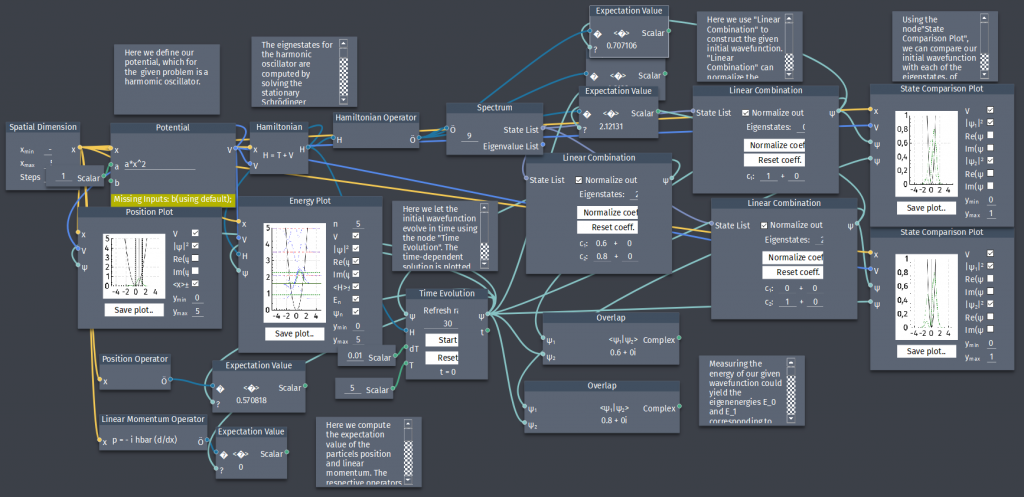# Linear combinations in the harmonic oscillator

A particle in the harmonic oscillator has initial wave function:

$\Psi(x,0)=A[3\psi_{0}(x)+4\psi_{1}(x)]$

a) Determine $A$ to normalize $\Psi(x,0)$.

b) Construct the time-dependent state $\Psi(x,t)$ and observe the overlap $\langle \Psi(x,t)|\Psi_{0}(x,0)\rangle$ and $\langle \Psi(x,t)|\Psi_{1}(x,0) \rangle$.

c) Compute $\langle x \rangle$ and $\langle p \rangle$.

d) Compute the energy of $\Psi(x,t)$.